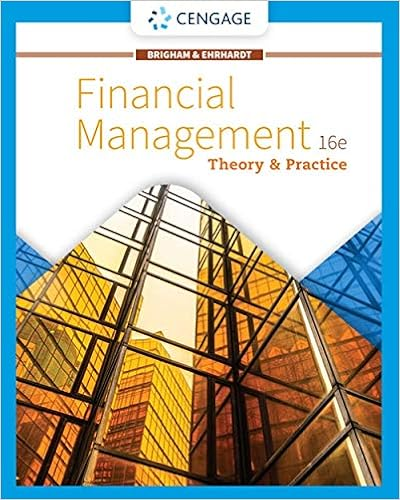Assume the following information us investors have

• Notes
• 31
• 94% (115) 108 out of 115 people found this document helpful

This preview shows page 21 - 23 out of 31 pages.

We have textbook solutions for you!
The document you are viewing contains questions related to this textbook.The document you are viewing contains questions related to this textbook.
Chapter 17 / Exercise 028
Financial Management: Theory & Practice
Brigham/EhrhardtExpert Verified
33. Assume the following information: U.S. investors have \$1,000,000 to invest: 1-year deposit rate offered by U.S. banks = 12% 1-year deposit rate offered on Swiss francs = 10% 1-year forward rate of Swiss francs = \$.62 Spot rate of Swiss franc = \$.60 Given this information: b. interest rate parity doesn't exist and covered interest arbitrage by U.S. investors results in a yield above what is possible domestically. 34. Assume the following information: Current spot rate of Australian dollar = \$.64 Forecasted spot rate of Australian dollar 1 year from now = \$.59 1-year forward rate of Australian dollar = \$.62 Annual interest rate for Australian dollar deposit = Annual interest rate in the U.S. = Given the information in this question, the return from covered interest arbitrage by U.S. investors with \$500,000 to invest is ____%. e. about 5.59 35. Assume the following bid and ask rates of the pound for two banks as shown below: Bid Ask Bank C \$1.61 \$1.63 Bank D \$1.58 \$1.60 As locational arbitrage occurs: d. the bid rate for pounds at Bank C will decrease; the ask rate for pounds at Bank D will increase. 36. Assume the bid rate of an Australian dollar is \$.60 while the ask rate is \$.61 at Bank Q. Assume the bid rate of an Australian dollar is \$.62 while the ask rate is \$.625 at Bank V. Given this information, what would be your gain if you use \$1,000,000 and execute locational arbitrage? That is, how much will you end up with over and above the \$1,000,000 you started with? 9% 6%
We have textbook solutions for you!
The document you are viewing contains questions related to this textbook.The document you are viewing contains questions related to this textbook.
Chapter 17 / Exercise 028
Financial Management: Theory & Practice
Brigham/EhrhardtExpert Verified
37. Assume the following information for a bank quoting on spot exchange rates: Exchange rate of Singapore dollar in U.S. \$ = \$.60 Exchange rate of pound in U.S. \$ = \$1.50 Exchange rate of pound in Singapore dollars = S\$2.6 Based on the information given, as you and others perform triangular arbitrage, what should logically happen to the spot exchange rates?
38. Bank A quotes a bid rate of \$.300 and an ask rate of \$.305 for the Malaysian ringgit (MYR). Bank B quotes a bid rate of \$.306 and an ask rate of \$.310 for the ringgit. What will be the profit for an investor who has \$500,000 available to conduct locational arbitrage?
39. Which of the following is an example of triangular arbitrage initiation?
•••